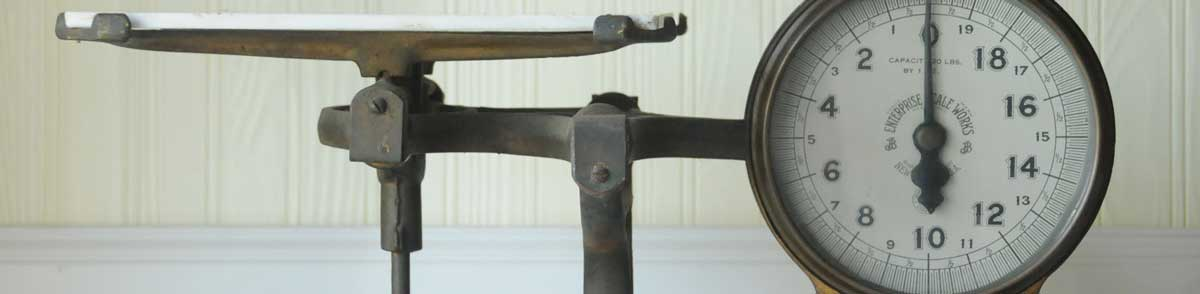# Kilograms to Carats Conversion

Enter the weight in kilograms below to get the value converted to carats.

Results in Carats:1 kg = 5,000 ct
Do you want to convert carats to kilograms?

## How to Convert Kilograms to CaratsTo convert a kilogram measurement to a carat measurement, multiply the weight by the conversion ratio. One kilogram is equal to 5,000 carats, so use this simple formula to convert:

carats = kilograms × 5,000

The weight in carats is equal to the kilograms multiplied by 5,000.

For example, here's how to convert 5 kilograms to carats using the formula above.
5 kg = (5 × 5,000) = 25,000 ct

## Kilograms

The definition of the kilogram changed in 2019. One kilogram was previously equal to the mass of the platinum-iridium bar, known as the International Prototype of the Kilogram, which was stored in Sèvres, France.

The 2019 SI brochure now defines the kilogram using the Planck constant, and it is defined using the meter and second. It is roughly equal to the mass of 1,000 cubic centimeters of water.

The kilogram, or kilogramme, is the SI base unit for weight and is also a multiple of the gram. In the metric system, "kilo" is the prefix for 103. Kilograms can be abbreviated as kg; for example, 1 kilogram can be written as 1 kg.

## Carats

The carat is a unit of mass/weight equal to 200 milligrams.

A carat is sometimes also referred to as a metric carat. Carats can be abbreviated as ct, and are also sometimes abbreviated as CD. For example, 1 carat can be written as 1 ct or 1 CD.

## Kilogram to Carat Conversion Table

Kilogram measurements converted to carats
Kilograms Carats
0.001 kg 5 ct
0.002 kg 10 ct
0.003 kg 15 ct
0.004 kg 20 ct
0.005 kg 25 ct
0.006 kg 30 ct
0.007 kg 35 ct
0.008 kg 40 ct
0.009 kg 45 ct
0.01 kg 50 ct
0.02 kg 100 ct
0.03 kg 150 ct
0.04 kg 200 ct
0.05 kg 250 ct
0.06 kg 300 ct
0.07 kg 350 ct
0.08 kg 400 ct
0.09 kg 450 ct
0.1 kg 500 ct
0.2 kg 1,000 ct
0.3 kg 1,500 ct
0.4 kg 2,000 ct
0.5 kg 2,500 ct
0.6 kg 3,000 ct
0.7 kg 3,500 ct
0.8 kg 4,000 ct
0.9 kg 4,500 ct
1 kg 5,000 ct

## References

1. International Bureau of Weights and Measures, The International System of Units, 9th Edition, 2019, https://www.bipm.org/utils/common/pdf/si-brochure/SI-Brochure-9.pdf
2. National Institute of Standards and Technology, Precious Metals Conversion Information, https://www.nist.gov/pml/weights-and-measures/laws-and-regulations/precious-metals-conversion